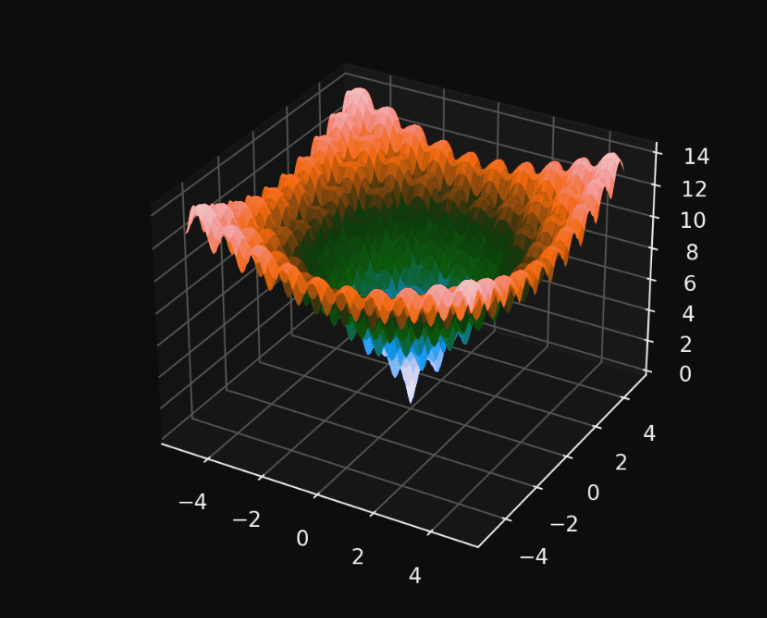## Introduction

Evolutionary computation uses differential evolution (DE) to optimize a problem by iteratively improving candidate solutions according to a given quality metric. Metaheuristics are methods that make very few assumptions about the problem to be solved and can search a very large space of potential solutions. Metaheuristics such as DE, however, do not guarantee that an optimal solution will ever be found.

Unlike gradient descent and quasi-newton methods which require the optimization problem to be differentiable, DE does not use the gradient of the problem being optimized. Therefore, DE can also be used for optimization problems that are not even continuous, noisy, or change over time.

As part of the optimization process, DE maintains a population of candidate solutions, combines them according to its simple formulae, and then keeps whichever candidate solution has the best score or fitness for the optimization problem at hand. In this way, the optimization problem is treated as a black box that merely provides a measure of quality given a candidate solution and the gradient is therefore not needed## The DE Algorithm

DE algorithms work by having a population of candidate solutions (called agents). By combining the positions of existing agents from a population, these agents are moved around in the search space.it is an improvement then it is accepted and forms part of the population, otherwise the new position is simply discarded. The process is repeated and by doing so it is hoped, but not guaranteed, that a satisfactory solution will eventually be discovered.

Formally, let

f:Rn —>R   be the fitness function which must be minimized.

The function takes a candidate solution as an argument in the form of a vector of real numbers and produces a real number as output which indicates the fitness of the given candidate solution. The gradient of f is not known. The goal is to find a solution m for which f(m)f(p) for all p in the search-space, which means that m is the global minimum.

Let x ∈ Rn designate a candidate solution (agent) in the population. The basic DE algorithm can then be described as follows

Choose the parameters

NP is the population size, i.e. the number of candidate agents or “parents”; a typical setting is 10n

The parameter CR ∈ [0,1]  is called the crossover probability and the parameter F ∈ [0,2] is called the differential weight. Typical settings are F=0.8 and CR=0.9

• Optimization performance may be greatly impacted by these choices; see below.
• Initialize all agents x with random positions in the search-space.
• Until a termination criterion is met (e.g. number of iterations performed, or adequate fitness reached), repeat the following:
• For each agent x in the population do

• Pick three agents a, b and c from the population at random, they must be distinct from each other as well as from agent X. (a is called the “base” vector.)
• Pick a random index R ∈ {1,…..,n} where n  is the dimensionality of the problem being optimized.
• Compute the agent’s potentially new position Y=[y1,…..,yn]    as follows:
• For each i ∈ {1,…..,n} pick a uniformly distributed random number Ri ∼ U(0,1)
• If Ri < CR or i = R then set yi = ai + F X (bi – ci ) otherwise set yi = xi (Index position R is replaced for certain.)
• If f(y) ≤ f(x) then replace the agent xin the population with the improved or equal candidate solution Y.
• Pick the agent from the population that has the best fitness and return it as the best found candidate solution.## Implementation part

Use %use S2 or else your code might not work
Here as you can see we have a objective function to minimize

				
// the objective function to minimize
%use s2
val f: RealScalarFunction = object : AbstractBivariateRealFunction() {
override fun evaluate(x: Double, y: Double): Double {
return (x - 1) * (x - 1) + (y - 3) * (y - 3) // (x - 1)^2 + (y - 3)^2
}
}



Here we have created a problem of integer programming and then assigned a random number generator.

				
// construct an integer programming problem
val problem: IPProblem
// both x and y need to be integers
= IPProblemImpl1(f, null, null, intArrayOf(1, 2), 0.0)

// a uniform random number generator
val rng: RandomLongGenerator = UniformRNG()
rng.seed(123456798L)



Here we have created an instance using generic algorithm for out DE(Differential Evolution) by initializing random number generator, parameters, number of iterations, etc.

				
// construct an instance of a genetic algorithm solver
val solver = DEOptim(
object : DEOptim.NewCellFactory {
override fun newCellFactory() : DEOptimCellFactory {
return IntegralConstrainedCellFactory(
Rand1Bin(0.5, 0.5, rng), // the DE operator
IntegralConstrainedCellFactory.SomeIntegers(problem))
}
},
rng, // a uniform random number generator
1e-15, // a precision parameter
100, // the maximum number of iterations
20 // the maximum number of iterations of no improvement
)



				
val soln: IterativeSolution = solver.solve(problem)
val xmin: Vector = soln.search(
// the boundaries: [-10, 10], [-10, 10]
DenseVector(-10.0, 10.0),
DenseVector(10.0, -10.0),
DenseVector(10.0, 10.0),
DenseVector(-10.0, -10.0)
)


				
val fxmin: Double = f.evaluate(xmin) // the mimimum
println("Differential Evolution")
println(String.format("f(%s) = %f", xmin, fxmin))



## Conclusion

Differential evolution algorithms were faster in most situations when it came to convergence speed. Population-based optimization methods are differential evolution algorithms. In other words, they seek to minimize the cost function by involving whole generations of individuals.